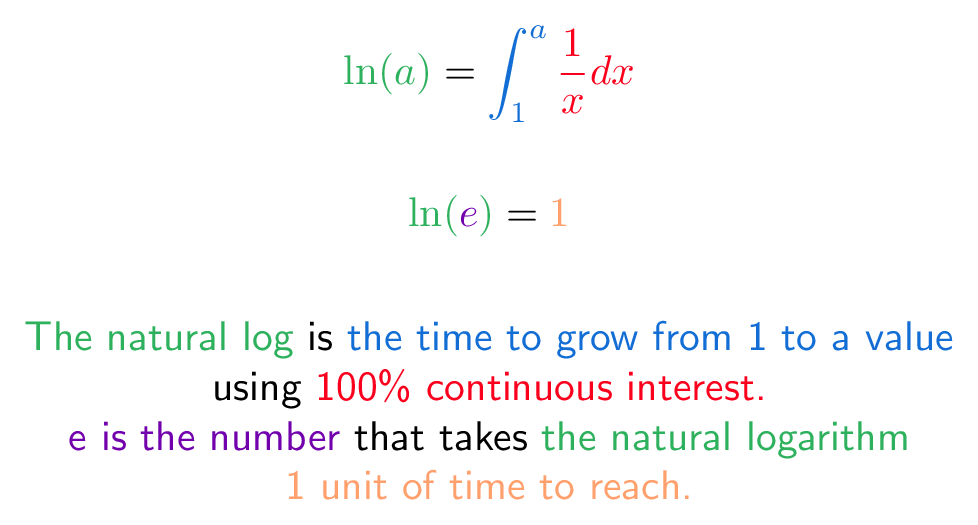# # E (Mathematical Constant 4)

## # Colorized Definition\newcommand{\growth}{\color{c1}}
\newcommand{\naturallog}{\color{c2}}
\newcommand{\unitInterest}{\color{c3}}
\newcommand{\timetogrow}{\color{c4}}
\newcommand{\unitTime}{\color{c5}}

$$\naturallog \ln(a) \plain = \timetogrow \int _{1}^{a} \unitInterest {\frac {1}{x}} dx$$
$$\naturallog \ln( \growth{e} \naturallog ) \plain = \unitTime 1$$

\naturallog The natural log
\plain is
\timetogrow the time to grow from 1 to a value
\\
\plain using
\unitInterest 100\% continuous interest.
\\
\growth e is the number
\plain that takes
\naturallog the natural logarithm
\\
\unitTime 1 unit of time to reach.